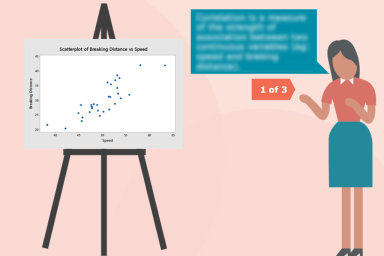Interactive

# Correlation and Regression

100% Effective
Updated Jul 07, 2020

In this course, you will learn what correlation is and how it is measured, what regression is and how it creates the regression model, how the quality of the regression model is examined, and how correlation and regression are used in Lean Six Sigma.

Explain what correlation is and how it is measured. Explain what regression is and how it creates the regression model (or transfer function). Explain how the quality of the regression model is examined by means of residual plots. Explain how correlation and regression are used in Lean Six Sigma.

;